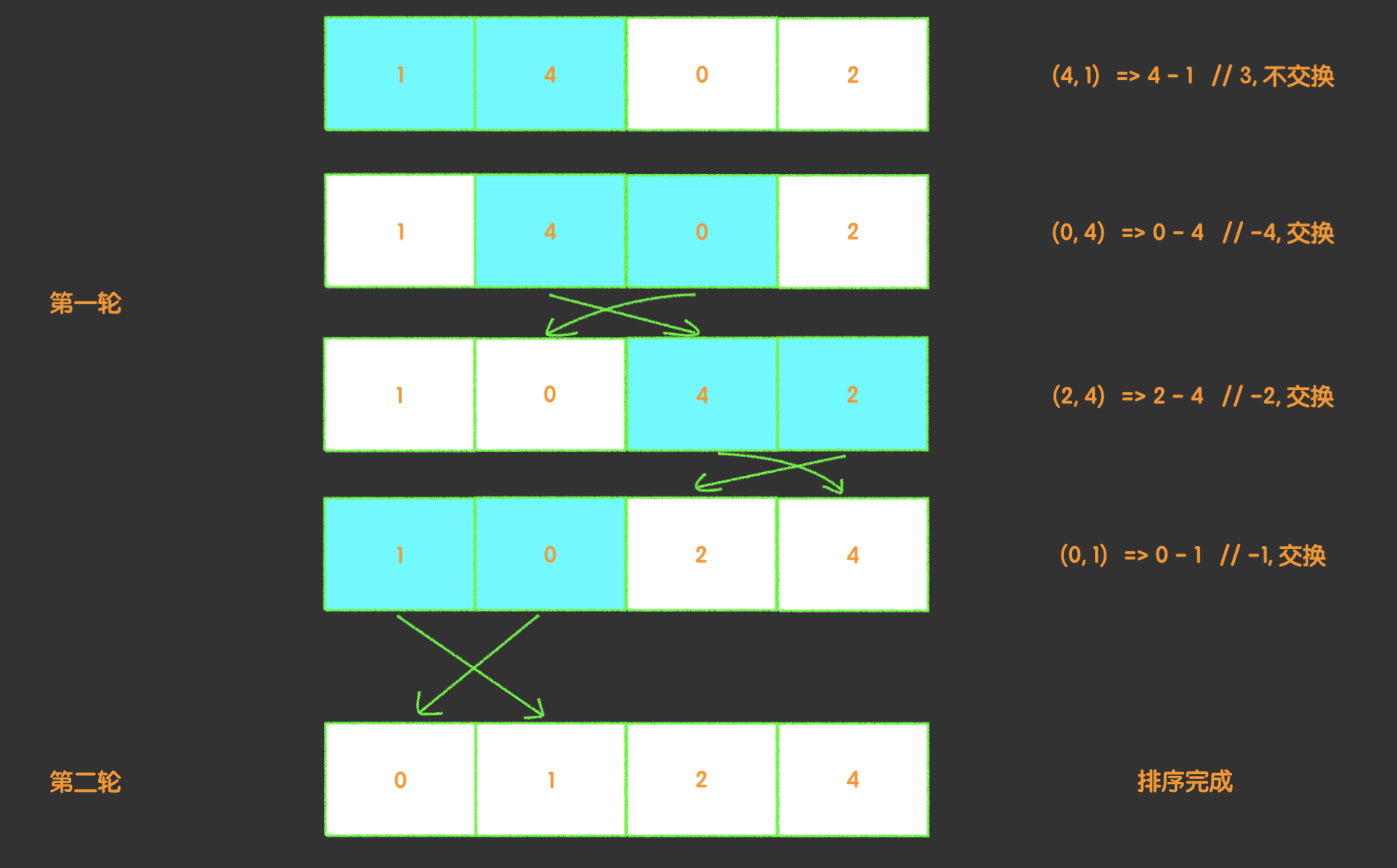``````输入: [0,1,0,3,12]

``````

• 必须在原数组上操作，不能拷贝额外的数组。

• 尽量减少操作次数。

``````const  format = (arr) => arr.sort((a, b) => b === 0 ? -1 : 1)
``````

``````const  format = (arr, target) => arr.sort((a, b) => b === target ? -1 : 1)
``````